Circumcenter Worksheet Name: ANSWER KEY Date: ______________ 1. a. Plot the following points: (0,8); (-8,-6); and (8,-6) b. Construct line segments between the points to form a triangle. c. Construct perpendicular bisectors to find the circumcenter of the triangle. d. Draw a circle around the triangle which falls on each of the plotted points. e. Draw a star at the circumcenter. f. Is the triangle an equilateral triangle, a right triangle, or a scalene triangle?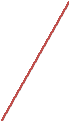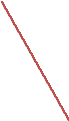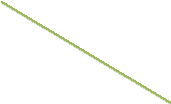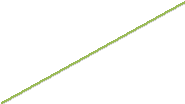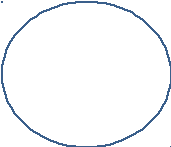Equilateral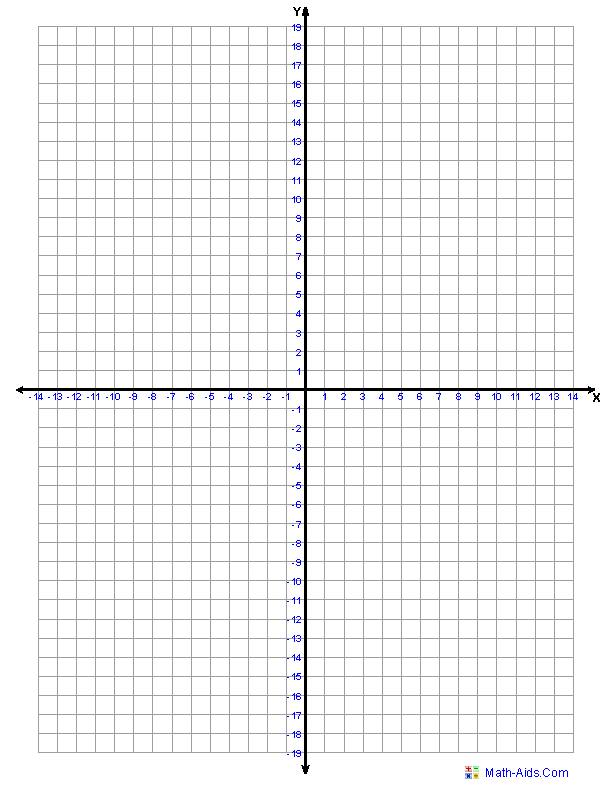2. a. Plot the following points: (-6,11); (9,11); and (-6,-10) b. Construct line segments between the points to form a triangle. c. Construct perpendicular bisectors to find the circumcenter of the triangle. d. Draw a circle around the triangle which falls on each of the plotted points. e. Draw a star at the circumcenter. f. Is the triangle an equilateral triangle, a right triangle, or a scalene triangle?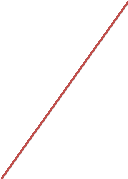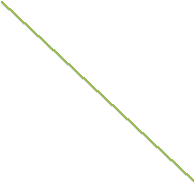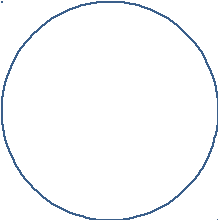Right3. a. Plot the following points: (-8,4); (7,16); and (-6,-8) b. Construct line segments between the points to form a triangle. c. Construct perpendicular bisectors to find the circumcenter of the triangle. d. Draw a circle around the triangle which falls on each of the plotted points. e. Draw a star at the circumcenter. f. Is the triangle an equilateral triangle, a right triangle, or a scalene triangle? Scalene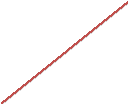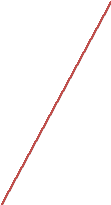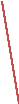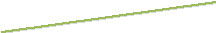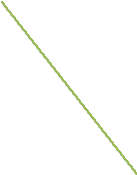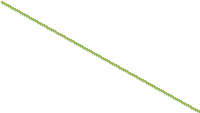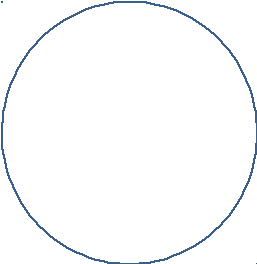4. Write a paragraph to compare/contrast the circumcenters of equilateral triangles, right triangles, and scalene triangles. Answers will vary. The main idea is that the circumcenter will fall inside the equilateral triangle and outside of the scalene triangle. But for the right triangle, it will fall on the midpoint of the hypotenuse.Share with your friends: